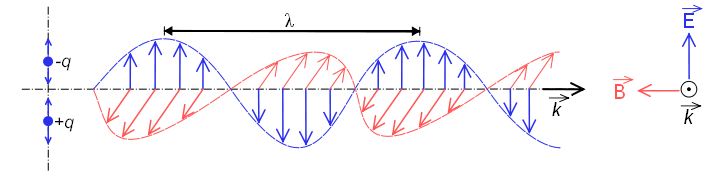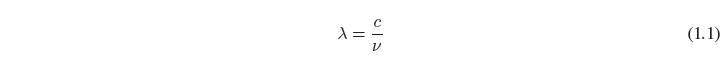Electromagnetic waves are formed by electric and magnetic fields that oscillate perpendicular to each other and perpedicular to the direction of propagation of the wave. Electromagnetic radiation propagates at the speed of $2,998\times 10^8\;m/s$, coinciding with the speed of light.In this figure you can see an electromagnetic wave polarized in the plane (the vectors of the electric field are contained in the same plane). This type of wave is produced by the oscillation of an electric charge moving in a straight line. The radiation emitted by atoms or molecules when heated does not present polarization, due to the random movement that they present.

Wavelength is called $\lambda$ to the distance between two successive crests, this part of the wave is called cycle.

Frequency $\nu$ is called the number of cycles that pass through a point in the unit of time.

The relationship between wavelength and frequency is:where $c$ represents the speed of light.

The inverse of the wavelength is called the wave number:The wave number is usually expressed in $cm^{-1}$, it represents the number of wave cycles in a centimeter and is widely used in infrared spectroscopy.